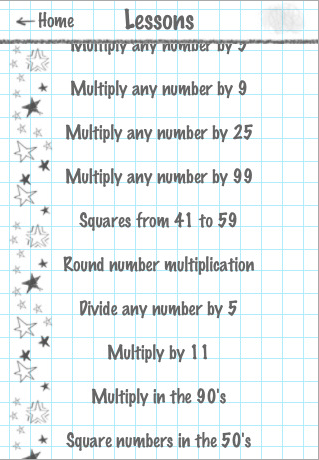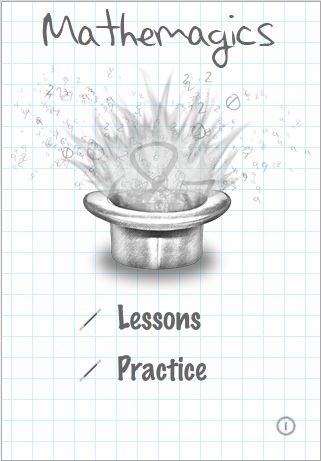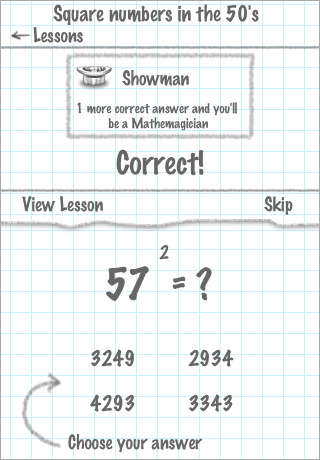December 10, 2023# Blue Lightning Labs Announces Mathemagics 1.0

Posted March 5, 2009 at 2:14pm by iClarified · 3579 views
Blue Lightning Labs has announced their new iPhone application Mathemagics, an application for learning to mentally calculate math problems at phenomenal speeds. Mathemagics contains 15 different math tricks for multiplying, dividing and squaring numbers faster than many people can use a calculator. Aspiring Mathemagicians learn and then practice any of the 15 math tricks while progressively moving to higher levels of proficiency.

Mathemagics is perfect for students, teachers, or anyone else looking to kick their brain function into high gear. The applications hand-drawn look engages the user and is well-suited for quick practice sessions. Users are challenged to achieve new levels of competence, from apprentice to mathemagician, for each trick.

"Learning to calculate math mentally helps train your brain to think efficiently," said Shane Crawford of Blue Lightning Labs. "I think it helps give me an edge in situations where others might fumble with a calculator. Mathemagics is perfect for quick training sessions and it gives me a mental pick-me-up when I need it."

Mathemagics 1.0 challenges users to master 15 math tricks:
* Multiply as high as 20 x 20
* Square numbers ending in 5
* Square any two digit number
* Square numbers from 41 to 59
* Square numbers in the 50's
* Doubling and Halving
* Quickly multiply by 4
* Quickly multiply by 5
* Quickly multiply by 9
* Quickly multiply by 11
* Quickly multiply by 25
* Quickly multiply by 99
* Multiply numbers in the 90's
* Round number multiplication
* Quickly divide by 5

Pricing and Availability:
Mathemagics is available now on the iTunes App Store for just \$2.99 (USD).